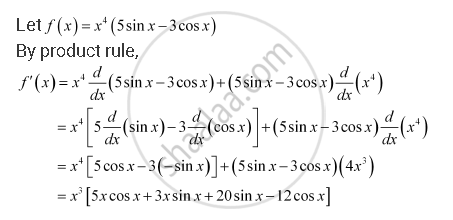CBSE (Science) Class 11CBSE
Share
Notifications

View all notifications
Books Shortlist
Your shortlist is empty

# Solution for Find the Derivative of the Following Functions (It is to Be Understood that A, B, C, D, P, Q, R and S Are Fixed Non-zero Constants and M and N Are Integers): X4 (5 Sin X – 3 Cos X) - CBSE (Science) Class 11 - Mathematics

Login
Create free account

Forgot password?
ConceptDerivative Algebra of Derivative of Functions

#### Question

Find the derivative of the following functions (it is to be understood that abcdp, q, r and s are fixed non-zero constants and m and n are integers): x4 (5 sin x – 3 cos x)

#### SolutionIs there an error in this question or solution?

#### APPEARS IN

NCERT Solution for Mathematics Textbook for Class 11 (2013 to Current)
Chapter 13: Limits and Derivatives
Q: 22 | Page no. 318
Solution Find the Derivative of the Following Functions (It is to Be Understood that A, B, C, D, P, Q, R and S Are Fixed Non-zero Constants and M and N Are Integers): X4 (5 Sin X – 3 Cos X) Concept: Derivative - Algebra of Derivative of Functions.
S